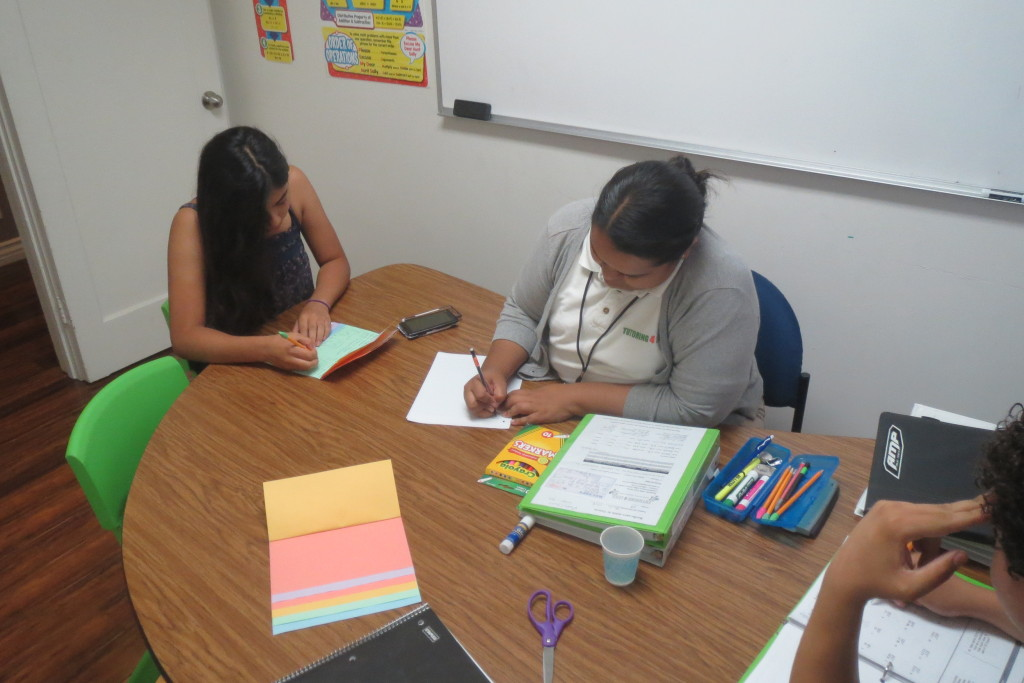#The Tutoring 4 Less approach to trigonometry involves trigonometry tutoring that’s in your student’s corner, giving them the support that will support their individual learning style and put them on the right path for academic success. Solving triangles is one of the many aspects of trigonometry that students study in high school. Tutoring 4 Less helps your student to develop the methods needed to solve triangles using trigonometric functions and identities. The study of the properties of these functions and related applications form the subject of trigonometry. Trigonometry has applications in navigation, surveying, construction, and many other branches of science, including mathematics and physics. We assist our students in realizing the practical applications of trigonometry, and other branches of mathematics, so that they can understand the importance of these subjects in everyday life.

## Online Tutoring For Trigonometry

Not all teaching experiences are the same. Some high school students get great benefit out of in-class support from their math teachers. Others may struggle with that kind of environment, and blossom best with private trigonometry tutoring, whether in-person or online. Tutoring 4 Less provides options for students who want additional academic support with trigonometry to take online trigonometry tutoring sessions with our expert staff. Experience tutoring online and see how it helps your understanding of the topic!

## One-to-One Trigonometry Tutoring

Trigonometry is the branch of mathematics that studies the relationships between lengths and angles of triangles. Historically, trigonometry was developed to help find the measurements in triangles as an aid in navigating and surveying of land. Today, it is used to define the relations between the known or unknown elements of a triangle or other geometric shapes. In a triangle, there are six basic elements; 3 sides and 3 angles. This branch of mathematics is mostly associated with planar right-angle triangles, which are mostly two-dimensional triangles with one angle measurement equal to 90 degrees. Tutoring 4 Less tutors approach the subject from a student-centered perspective. We find that students learn math best when the teaching style of our private tutors complements their learning style. All of our high school level math tutoring sessions follow this same approach, from Trigonometry to Pre-calculus. One-to-one trigonometry tutoring can be the difference between struggling with trigonometry, and advancing to honors and AP programs.Topics that our trigonometry program covers are:

• Review of Algebra and Geometry
• Rectangular Coordinates and their application to graph and circles
• Functions
• Trigonometric Functions & the Unit Circle
• Angles and Their Measures
• Properties of the Trigonometric Functions
• Right Angle Trigonometry
• Graphing the 3 Trigonometric Functions and their Inverses
• Trigonometric Identities
• Applications of the Trigonometric Identities and Functions